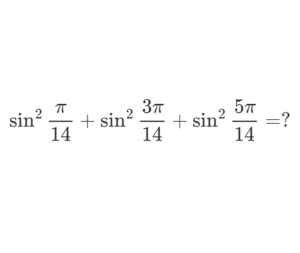Home -> Solved problems -> Prove the following equality

## Prove the following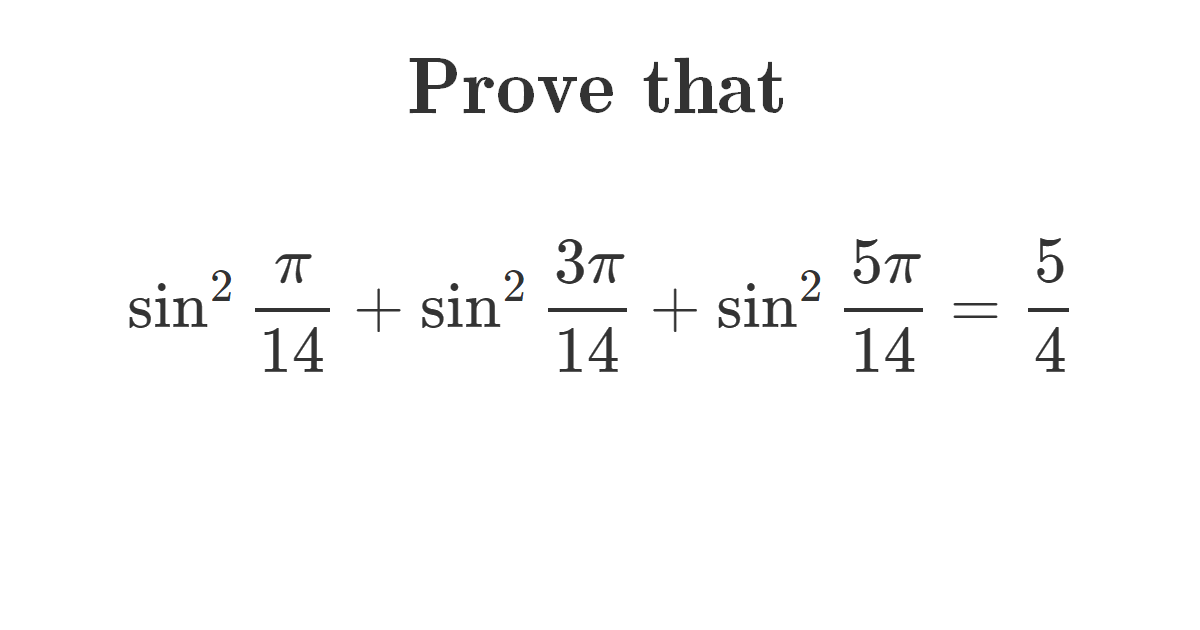### Solution

Let $$f$$ be a function such that for $$x \in \mathbb{R}: f(x)=\cos 2 x+\cos 6 x+\cos 10 x$$
\begin{aligned} &2 \sin 2 x f(x)=2 \sin 2 x \cdot \cos 2 x+2 \sin 2 x \cdot \cos 6 x+2 \sin 2 x \cos 10 x \\\\ &=\sin 4 x+\sin (2 x+6 x)+\sin (2 x-6 x)+\sin (2 x+10 x)+\sin (2 x-10 x) \\\\ &=\sin 4 x+\sin 8 x-\sin 4 x+\sin 12 x-\sin 8 x \\\\ &=\sin 12 x \end{aligned}
$\text { Let } x=\frac{\pi}{14}: 2 \sin \frac{2 \pi}{14} \cdot f\left(\frac{\pi}{14}\right)=\sin \frac{12 \pi}{14}$
\begin{aligned} &\Leftrightarrow 2 \sin \frac{\pi}{7} f\left(\frac{\pi}{14}\right)=\sin \frac{6 \pi}{7} \\\\ &\Leftrightarrow f\left(\frac{\pi}{14}\right)=\frac{\sin \frac{6 \pi}{7}}{2 \sin \frac{\pi}{7}}=\frac{\sin \left(\pi-\frac{\pi}{7}\right)}{2 \sin \frac{\pi}{7}}=\frac{\sin \frac{\pi}{7}}{2 \sin \frac{\pi}{7}}=\frac{1}{2} \end{aligned}
\begin{aligned} \sin ^{2} \frac{\pi}{14}+\sin ^{2} \frac{3 \pi}{14}+\sin ^{2} \frac{5 \pi}{14} &=\frac{1-\cos \frac{2 \pi}{14}}{2}+\frac{1-\cos \frac{6 \pi}{14}}{2}+\frac{1-\cos \frac{10 \pi}{14}}{2} \\\\ =& \frac{3-\left(\cos \frac{\pi}{7}+\cos \frac{3 \pi}{7}+\cos \frac{5 \pi}{7}\right)}{2}=\frac{3-f\left(\frac{\pi}{14}\right)}{2} \\\\ &=\frac{3-\frac{1}{2}}{2}=\frac{5}{4} \end{aligned}
Home -> Solved problems -> Prove the following equality

### Related Topics

Find the volume of the square pyramid as a function of $$a$$ and $$H$$ by slicing method.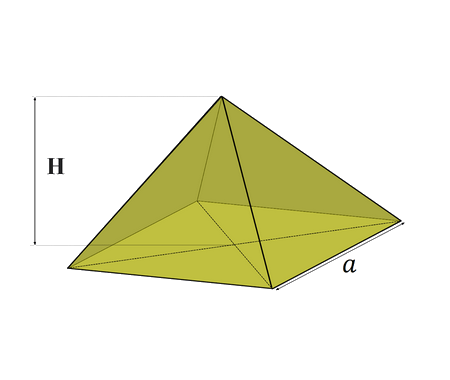Prove that $\lim_{x \rightarrow 0}\frac{\sin x}{x}=1$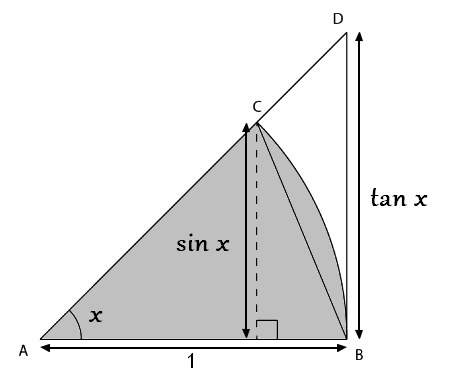Prove that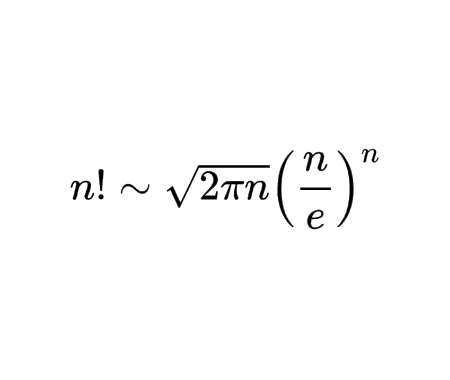Calculate the half derivative of $$x$$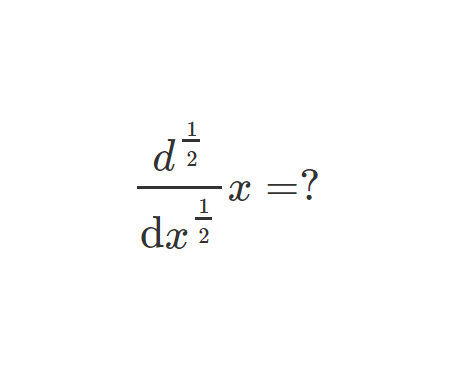Prove Wallis Product Using Integration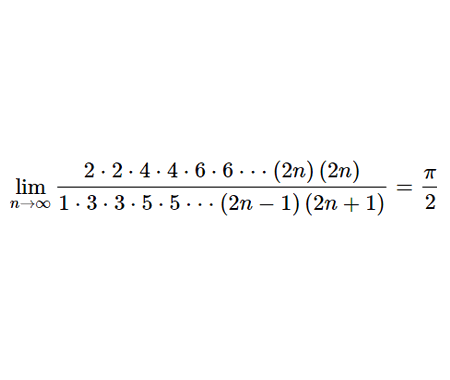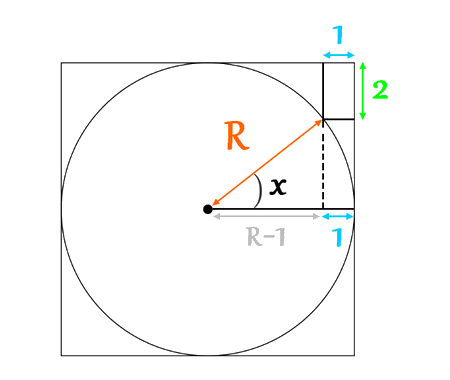Calculate the volume of Torus using cylindrical shells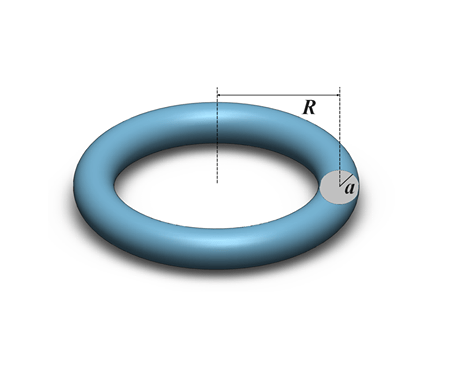Find the derivative of exponential $$x$$ from first principles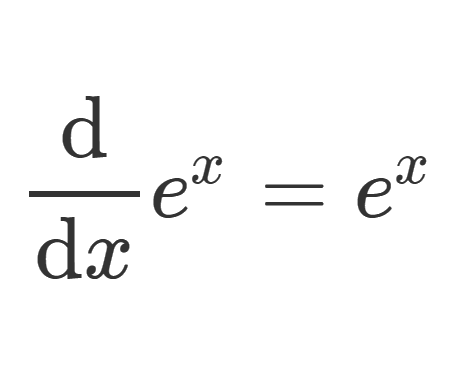Calculate the sum of areas of the three squares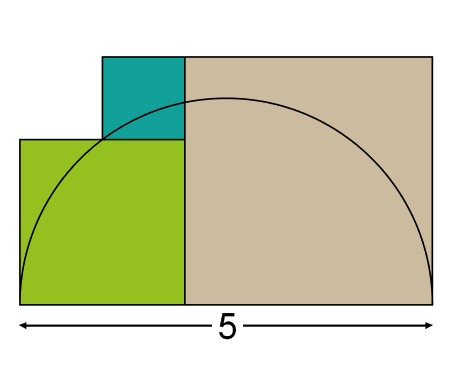Find the equation of the curve formed by a cable suspended between two points at the same height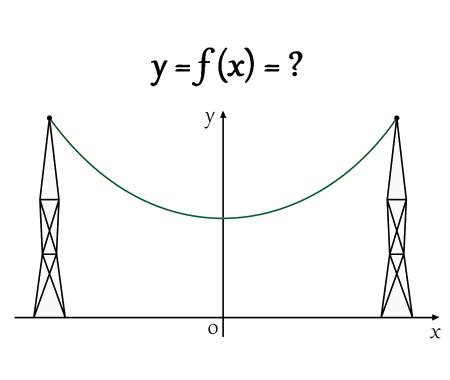Solve the equation for real values of $$x$$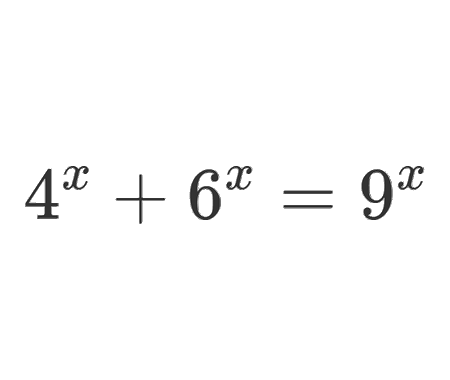Solve the equation for $$x\epsilon\mathbb{R}$$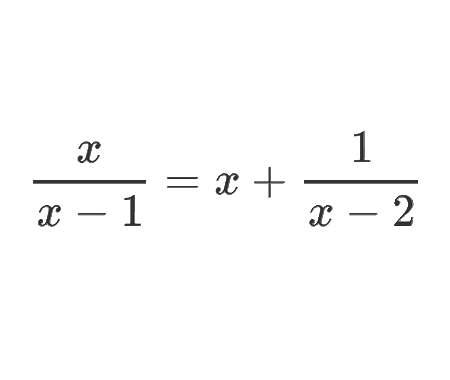Determine the angle $$x$$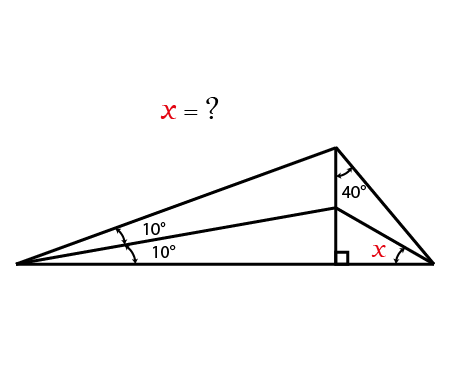Calculate the following limit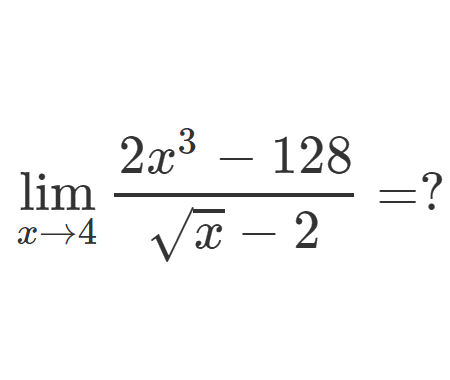Calculate the following limit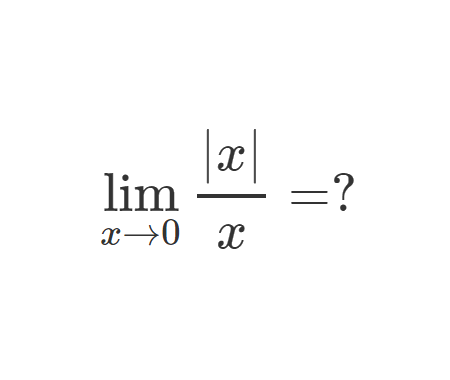Calculate the integral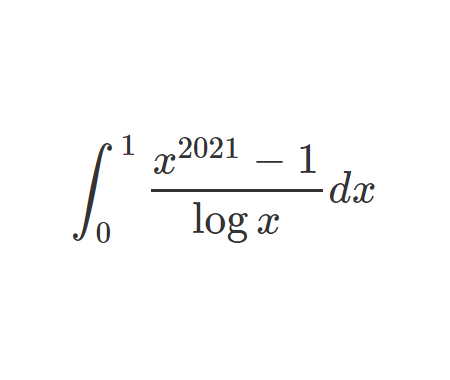Challenging problem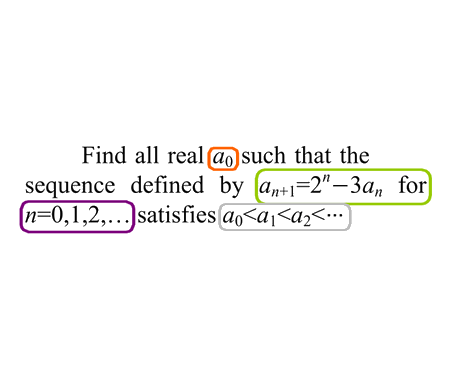Prove that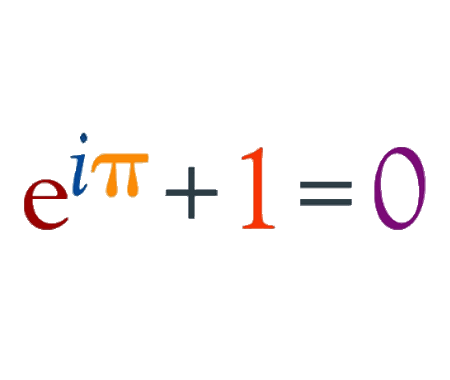Prove that $$e$$ is an irrational numberFind the derivative of $$y$$ with respect to $$x$$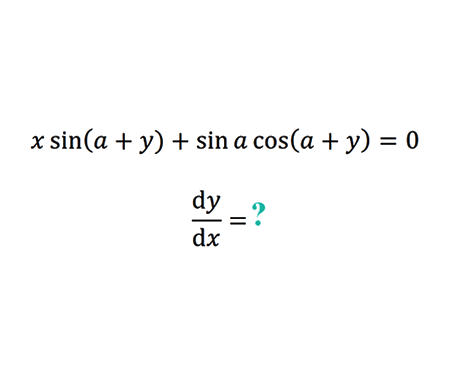Find the limit of width and height ratio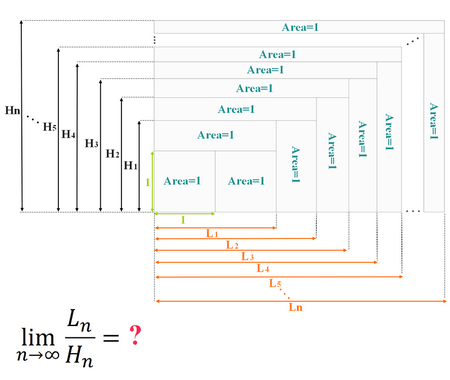How Tall Is The Table ?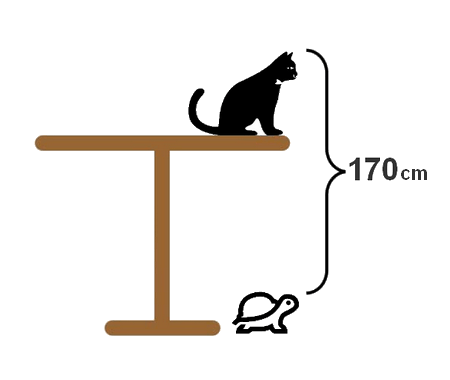Why 0.9999999...=1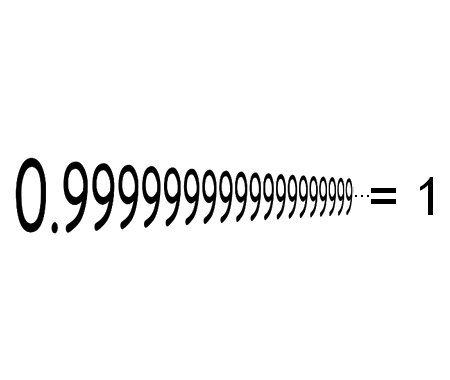Solve the equation for $$x \in \mathbb{R}$$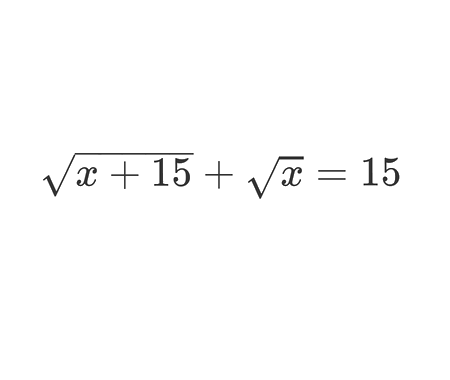Calculate the following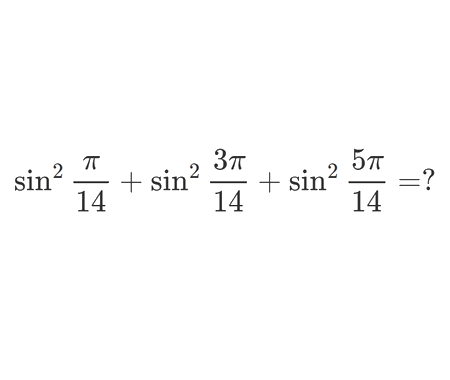Home -> Solved problems -> Prove the following equality

#### Share the solution: Prove the following equality